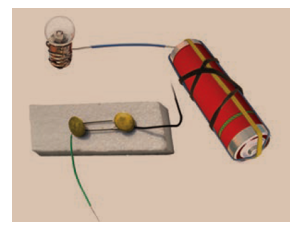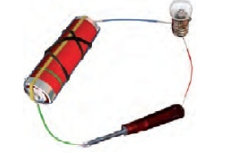# Electricity and Circuits Extra Questions

In this page we have Chapter 12 Electricity and Circuits Extra Questions . Hope you like them and do not forget to like , social share and comment at the end of the page.
Question 1
1. Complete the drawing shown below to indicate where the free ends of the two wires should be joined to make the bulb glow2. Why do electrician wear rubber hand gloves while working with electricity?
3. What are the advantages of a dry cell?
4. Why we should not operate electric switches with wet hands?
5. Using the “conduction tester” on an object it was found that the bulb begins to glow. Is that object a conductor or an insulator? Explain.
6. Why Electric wires are made of copper?

Question 2
1. What are the essential components of an electric circuit?
2. What is a conductor? Give two examples.
3. What alternative devices can we use if there is a sudden breakdown of electric supply?
4. Why do bulbs have two terminals?
5. What is current?
6. How can you generate electricity from sunlight?
7. Mention three drawbacks of voltaic cell?
8. Name two sources of electric current.
9. What is meant by battery?
10. What is the function of the switch in an electric circuit?
11. Why is distilled water used in the batteries and not the tap water?
12. What is the purpose of using an electric switch? Name some electrical gadgets that have switches built into them.

13. Question 3
(a)Differentiate between conductors and insulators.

(b)
(i)What is an insulator? Give two examples
(ii)Give one activity to prove that air is an insulator.
(iii) Write few uses of insulators

(c)
i. Explain why the bulb would not glow in the arrangement shown in the figure below.
ii. What steps can you take to make it glow. Explain with diagram.### Practice Question

Question 1 What is $\frac {1}{2} + \frac {3}{4}$ ?
A)$\frac {5}{4}$
B)$\frac {1}{4}$
C)$1$
D)$\frac {4}{5}$
Question 2 Pinhole camera produces an ?
A)An erect and small image
B)an Inverted and small image
C)An inverted and enlarged image
D)None of the above